Standard collision examples Development of equations Elastic vs Inelastic
Index

Collision concepts

 HyperPhysics***** Mechanics R Nave
Go Back

# Head-on Elastic Collisions, Target at Rest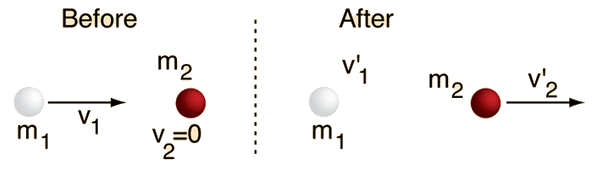Conservation of momentum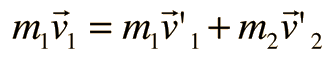Conservation of kinetic energy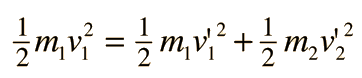To obtain expressions for the velocities after the collision, rewrite the above as: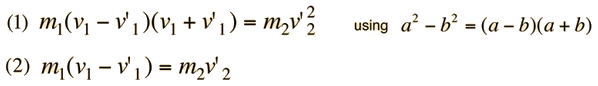Dividing these relationships gives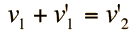which may be substituted into equation (2) above to obtain:Note that these equations apply only to the case where the target is at rest.

 Elastic collisions, target at rest Standard examples, elastic collisions Calculation for headon case.
Index

Collision concepts

 HyperPhysics***** Mechanics R Nave
Go Back

# Velocities After Collision

For head-on elastic collisions where the target is at rest, the derived relationshipmay be used along with conservation of momentum equationto obtain expressions for the individual velocities after the collision.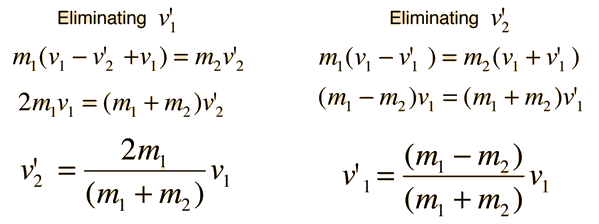These relationships may be used for any head-on collision by transforming to the frame of the target particle before using them, and then transforming back after the calculation. However, one may calculate the case for head-on collisions where both particles are moving with the relationships: